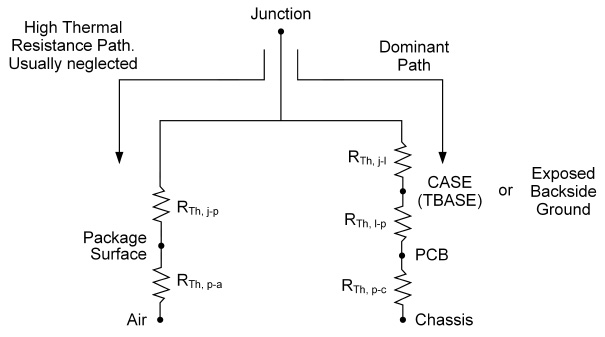#### 步骤1：计算器件的功耗（Pdiss）

如果已经知道你的Pdiss,  请在虚线下方输入。

Inputs
• Supply: Volts
• Current: mA
• Pin:
• Pout:
Outputs
• DC Power: Watts
• Gain: dB
• Drain/Collector Efficiency: %
• Power Added Efficiency: %
• Power Dissipated In Device (Pdiss): Watts
已经知道Pdiss值? Power Dissipated In Device (Pdiss): Watts

#### 步骤2：计算最大结温

Inputs
• Maximum Case (Tbase) Temperature: °C
• Rth Junction To Case: °C/Watt

注意:

测量值会因应用和测量方法不同而有所不同。 最准确的数据可在产品数据手册中查找。

Outputs
• Max Junction Temperature: °C

### Formulas Used

• DC Watts = Supply V × Current / 1000
• Gain (dB) = Pout dBm - Pin dBm
• Pin Watts = (10^(Pin dBm / 10)) / 1000
• Pout Watts = (10^(Pout dBm / 10)) / 1000
• Drain/Collector Efficiency % = (Pout Watts / DC Watts) × 100
• PAE % (Low Gain < 25dBm) = (Pout Watts - Pin Watts) x 100 / (DC Watts)
• Pdiss Watts = DC Watts + Pin Watts - Pout Watts

• Temperature Change °C = Rth Junction to Case × Pdiss Watts
• Max. Junction Temperature at max. Case Temp = Temp Change °C + max. Case Temp°C
• Note: Tbase = interface between part and PC board/heatsink.对使用此工具有任何疑问, 可发送电子邮件至Qorvo Tools团队，我们很乐意为您提供帮助。